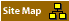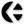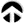The following Essay explores the mathematics of the Theory of Relativity and Quantum Theory. This text deals with the mental machinery - and machining - that mathematics represents.The Theory of Relativity can do what it does, well, because of its specific limitations; inherent in its underlying mathematics.The Quantum Theory (or the Theory of Probability), likewise, is crippled and conditioned by its mathematical foundation.By understanding the mathematical foundations, why they were used and what they represent, the limitations of the resulting construct (the science) can be better understood. In brief:Theory of Relativity posed a limit on the use of a model; use of the Lorentz equation tied the human observer in with that limitation.Quantum Theory addressed the involvement of the observer: use of the Schrödinger equation implied this.The mathematical machinery of the Theory of Relativity lies in the use of the Potential Equation: it defines a limit at any point the mathematician selects.The mathematical machinery of the Quantum Theory is found in the Series; the Taylor Series and use of multiple perspectives. In metaphor:The Theory of Relativity looked at reality using a mirror.The Quantum Theory looked at interactions using two mirrors. In an image: \//\ Relativity TheoryThe above image represents the reflecting boundary at the speed of light. /\\/ Probability TheoryThe above image represents the reflection between two atomic orbits. The Theory of Relativity is a way of looking at reflections.Compare it to looking in a mirror. \//\ Depending on where you stand, and position the mirror, you will see a difference between what you see directly, and what you see via that mirror.The Theory of Relativity positioned the mirror at the speed of light; this is an arbitrary placement. You can also place such a mirror at the speed of sound of - more relevant the speed of consciousness.The mirror technique helps out to identify in detail what happens for any motion close to that mirror. Because you cannot look beyond that mirror, whatever you will look at will seem to run into a wall: the barrier of Sound, the Barrier of Light, the Barrier of Consciousness, and so on.The so-called limitation of the Speed of life is not a fact; it is an assumption (later shown to be false; John Davidson, The Secret of the Vacuum)). It is the mathematical (psychological) tool of the insistent comparison with the speed of sound, or light, which seemingly fixes the perspective.Once you realise how this work you can change the perspective. You can place your 'mirror' at a different position; or you can even use multiple mirrors. Bill Tiller has proposed to used multiple mirrors, positioned at different angles at the same point, to be able to observe what take place at that point in more detail. The Theory of Relativity regarded the motion of planets, with respect of the (assumed) speed of light The Quantum Theory looked at the movement of electron between adjoining atomic orbits. By the assumption that the speed of light is fixed, it becomes a limit to what can be perceived by this method.By assuming that it is not possible to describe what happens between atomic orbits, this limits the model. Quantum Theory is a way of looking for repetitions.Compare it to looking using two mirrors. /\\/ In order to understand the Theory of Relativity, and Quantum Theory, look at the mathematics on which it is based. The Theory of Relativity uses a description of Lorentz, en Riemann. Quantum Theory used the description of Schrödinger and Taylor. The method for the Theory of Relativity is based on the inversion of perspective. It tries to get a clearer picture of the known, by comparing it to itself, in inverse.The method is Quantum Theory, the Theory of Probability, is based on pattern recognition by ‘successive elimination of the known’. Cauchy matrix sweeping, Taylor Series, Fourier Series, and Fourier Integrals are all examples of this approach. The Theory of Relativity in that sense is a general application of the notion “A = - (-A)”. (v - V(c))The Theory of Probability is essence applies the general principle of the concept “”. The principal ideas of the underlying models of mathematics will e summarised: Lorentz Transformation (v - vc) Riemann Transformation (loops within loops) mathematical Series (polynomials, Taylor, Fourier, Schrödinger) Cauchy Matrix Sweeping (eliminating the known) The mathematical Series is a mathematical fool for recognising patterns.It is based on the well known polynomial equation y = ax + bx^2 + cx^3 + ... and so on.Taylor and others realised that it basically meant that any value can be described as a combination of different functions.Another way to present this is: any point can be located by adding and subtracting graphs of different kind.Compare it to reaching any place on Earth by a combination of different means of transport. Cauchy matrix sweeping applies the same concept in the context of a Matrix. It identifies what is known in something unknown, and consistently taking that out of the equation to see what is left. Once you will have recognised all that was involved, the result will be zero. There is no difference between what you found (the unknown function), and what you created (a combination of known functions).[Welcome] [Core Concepts] [Topics] [Participants] [Publications] [Research] [Projects]